• Call Now

1800-102-2727•

# Convex mirror

Do you know which mirror is installed in your car as a side rearview mirror? In the car or vehicle, our requirement is to look at a wide area of the rear. Generally, we have three types of the mirror; plane mirror, concave mirror and convex mirror. All of them have different properties and hence different uses. In three of them, the convex mirror has the ability to make a virtual image of a small size. Hence we use a concave mirror in our cars so as to cover a larger rear area and to form a diminished image. Let's understand more about how it works!

Table of content

• Spherical mirror
• Convex mirror
• Characteristic of a convex mirror
• Terms related to Convex mirror
• Rule to draw a ray diagram
• Image formation by Convex mirror
• Mirror formula and magnification
• Uses of Convex mirror
• Practice problem
• FAQs

## Spherical mirror

Spherical mirrors are curved glass plates which are painted with a reflecting material like silver on one of the sides and the other side is used for the reflection. This spherical mirror can be of two types: one is a concave mirror and the other is a convex mirror. The spherical glass plates which are painted outward and the inner surface used for the reflection are the concave mirrors. The spherical glass plates which are painted from the inside and the outer surface used for the reflection are the convex mirrors.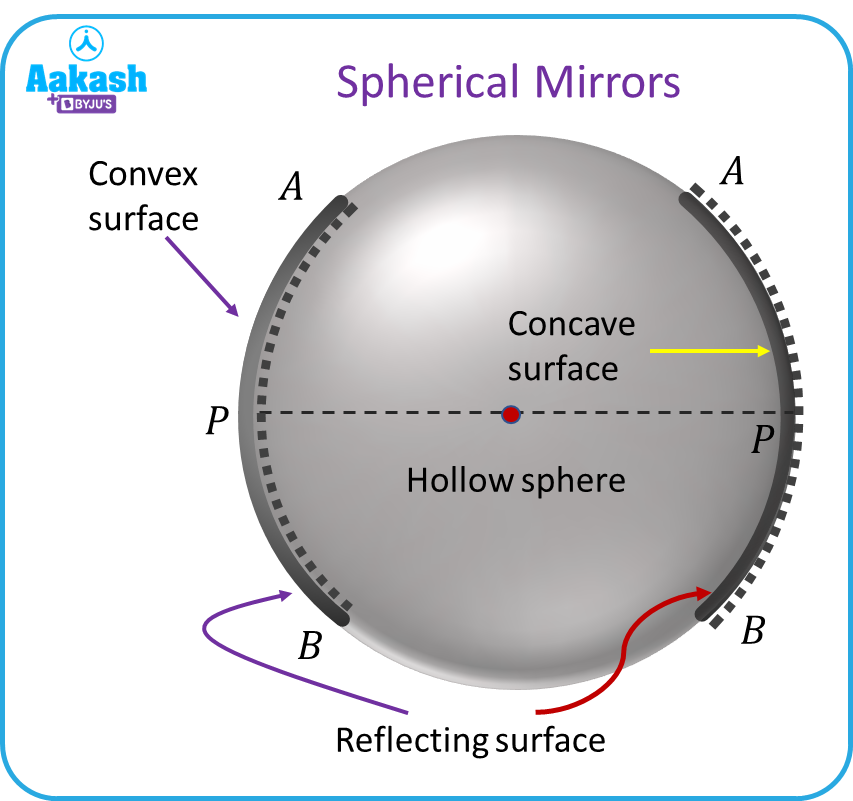## Convex mirror

The part of the hollow sphere which is silver painted from the inside and the outer surface is used for the reflection purpose and is known as a convex mirror. The figure shows a convex mirror.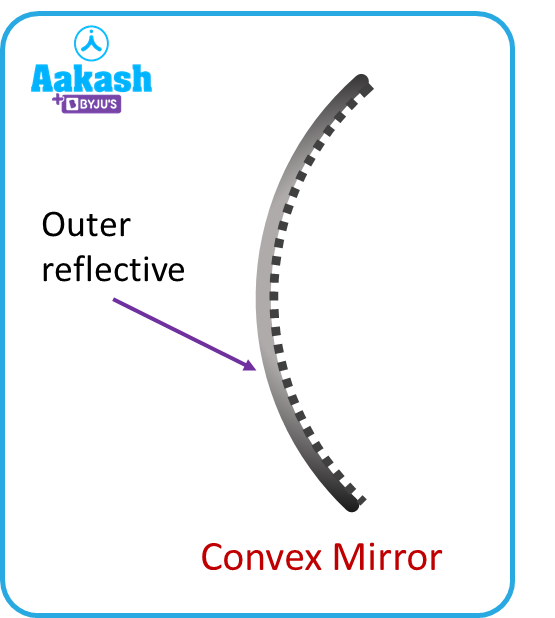## Characteristic of a convex mirror

A convex mirror has the following properties:

1. A convex mirror is also referred to as a diverging mirror because, when light rays hit its reflecting surface, they diverge.
2. Whatever the object's distance from the convex mirror, it always produces a virtual, erect and diminished image.
3. Convex mirrors do not focus the light ray toward a point as the rays after hitting always diverge.

## Term related to Convex mirror

These are the following terms related to the convex mirror.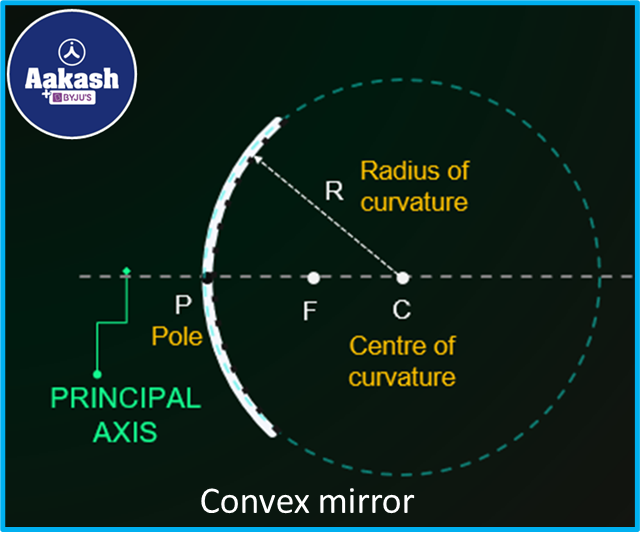1. Center of curvature - The centre of curvature is the centre of the imaginary sphere from which the convex mirror is taken. The centre of curvature is indicated by C as shown in the above figure.
1. Principal axis - A line joining the centre of curvature and the pole of the mirror is known as the principal axis.
1. Pole - Mirror pole refers to a point which is situated at the centre of the curved section of the mirror. It is denoted by P.
1. The radius of curvature - The radius of the imaginary sphere from which the convex mirror is taken is called the radius of curvature. The distance from any point on the spherical mirror to the centre of curvature is another way to define the radius of curvature. R is used to indicate it.
1. Focus - When a beam of parallel rays coming from infinity falls on the convex mirror, after the reflection it appears to pass through a point. This point is known as the focus of the spherical mirror. The letter F is used to denote it.
1. Focal length- The focal length of a spherical mirror is defined as the distance between the focus (F) and pole (P).

## Rule to draw a ray diagram

To trace the image of an object there are certain rules which help in locating the image.

1. When a ray of light parallel to the principal axis falls on a convex mirror, it seems to pass through the convex mirror's focus after the reflection as shown in the figure.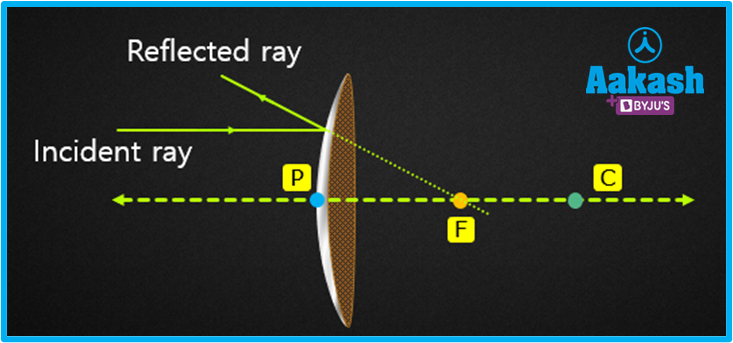1. When a ray is pointed in the direction of the focus and falls on a convex mirror, after the reflection it becomes parallel to the axis of the convex mirror as shown in the figure.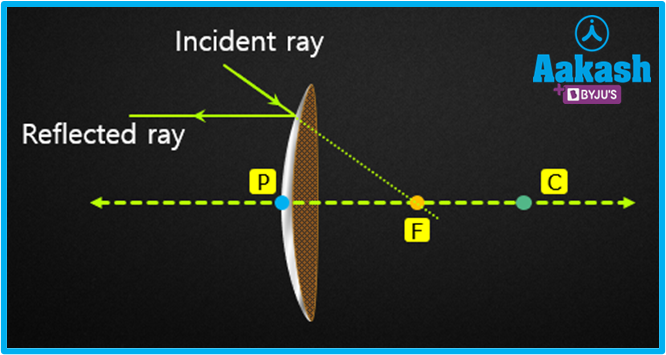1. When a ray of light falls on the convex mirror such that it is directed toward the centre of curvature of the mirror then after the reflection it returns back in the same direction.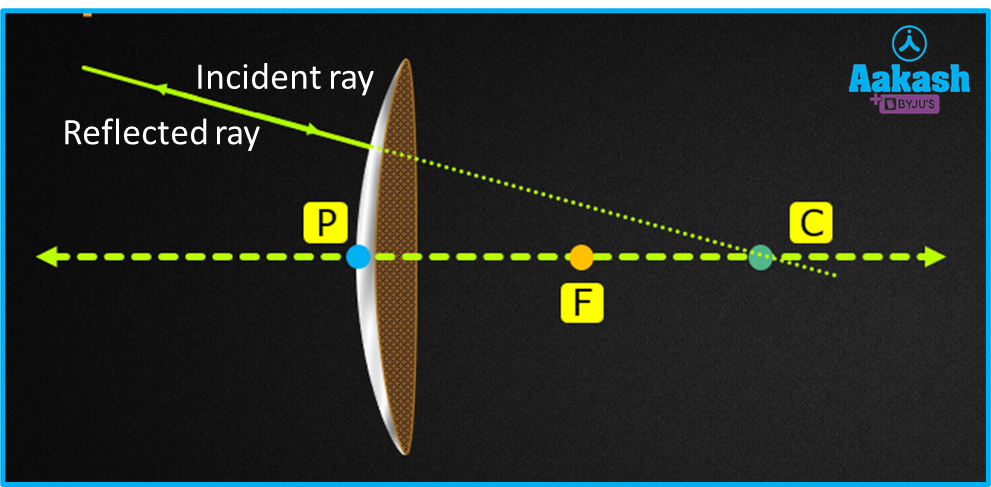1. When a ray incident on the pole of the mirror reflects obliquely similar to the plane mirror.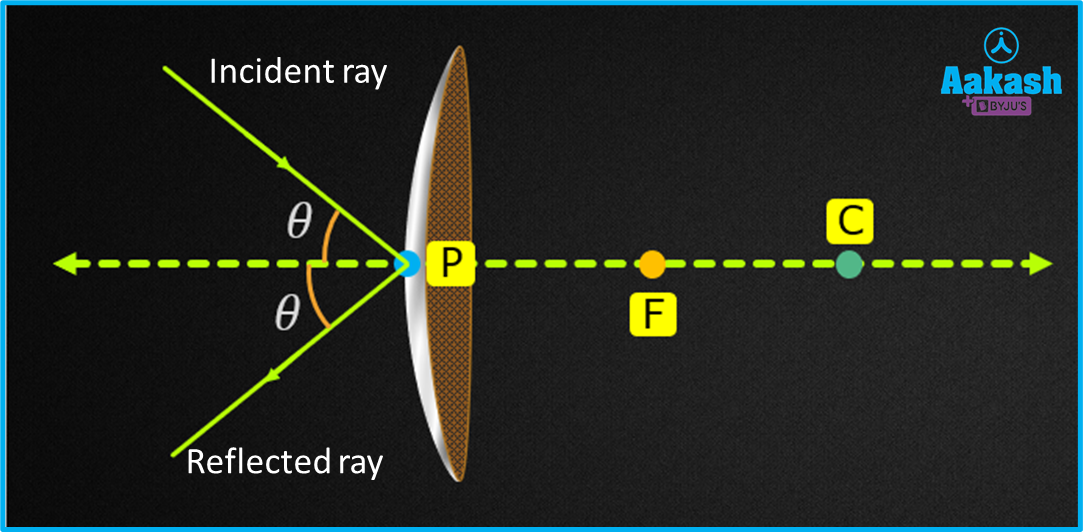## Image formation by Convex mirror

Regardless of the object's position, the image created by a convex mirror is always virtual and upright. Let's examine the types of images created by convex mirrors in this part. There are two possibilities for the placing of an object to get an image.

1. When an object is placed at infinity: For tracing the location of the image we need at least two light rays. Consider two parallel rays which are coming from infinity. After the reflection, they diverge and will never meet. If we extend these reflected rays behind the mirror they will meet somewhere in the focus of the mirror. Hence a virtual image is created at the focus when an object is positioned at infinity. In comparison to the object, the image is smaller in size.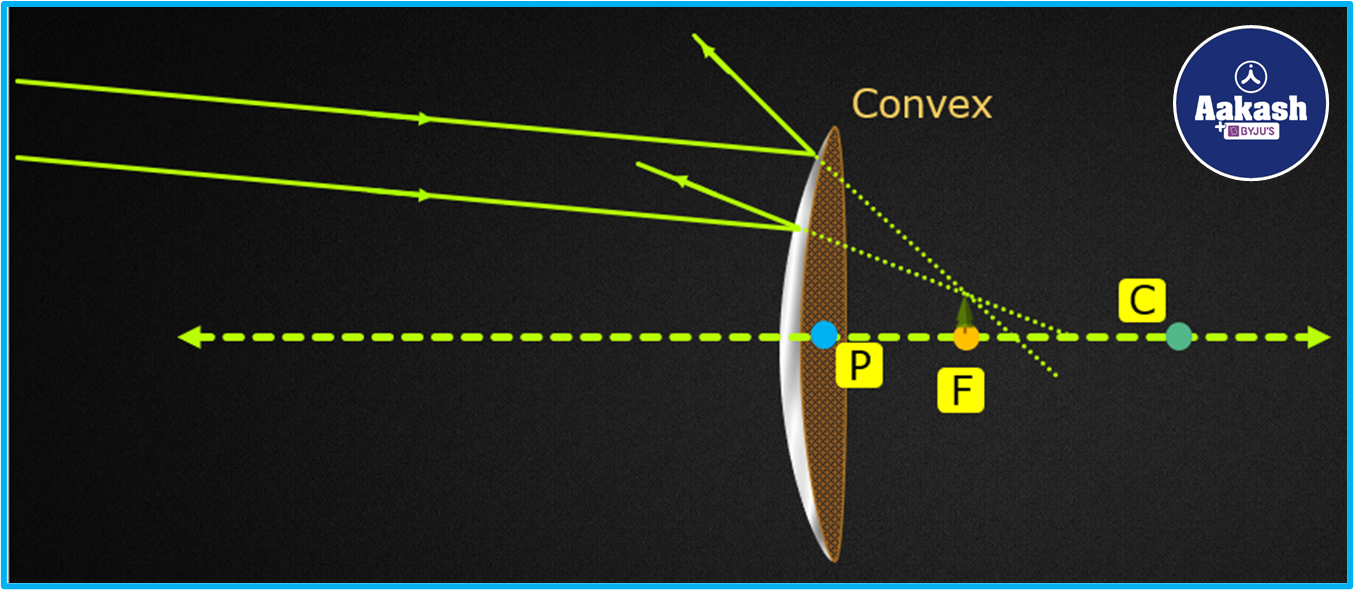1. When an object is placed between infinity and pole : Draw a ray parallel to the principal axis. After the reflection it will diverge in different directions. The ray directed towards the center of curvature will return in the same direction. If we extend these rays behind the mirror, a virtual and diminished image will form in between the pole and the focus of the mirror.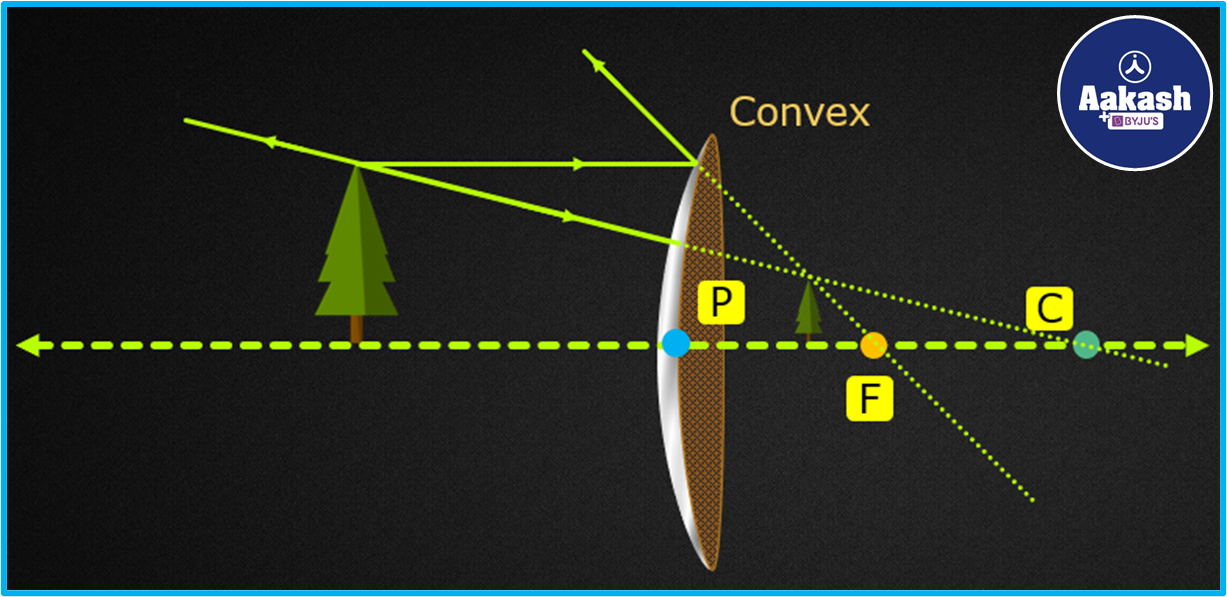## Mirror formula and magnification

The mirror formula is an equation that links focal length of the mirror, object distance and image distance. The equation is given as

$\frac{1}{f}=\frac{1}{u}+\frac{1}{v}$

Where,
u= The object's distance from the pole

v= The image's distance from the pole.

f= Focal length of the mirror

The height ratio of the image to the object is known as magnification. It is given as,

If u is the distance of the object from the mirror and v is the distance of the image then the magnification can be written as

$m=\frac{{h}_{i}}{{h}_{o}}=-\frac{v}{u}$

Sign convention : Distance measured in the direction of the incident ray is taken as positive and opposite to this direction is taken as negative. The distance in upward direction to the principal axis is taken as positive and the distance measured in downward direction is taken as negative.

## Uses of Convex mirror

The convex mirror can be used for the following purposes-

1. A convex mirror allows us to see a greater area which we could not do in a plane mirror.
2. In many cars, convex mirrors are used as rear-view (wing) mirrors. Convex mirrors are frequently used on the sides of cars to allow the driver to observe traffic or cars approaching from behind.
3. They are used on roadways to protect motorcyclists and drivers from danger in blind places like curves and corners.
4. They are used to provide a wider field of view. Convex mirrors are installed in building halls.

## Practice Problem

Q1. When an object is positioned 40 cm in front of a mirror, a virtual image appears at 20 cm behind the mirror. Determine the kind and focal length of the mirror.

Answer. Given, the distance of object u= -40 cm

Distance of image v=20 cm

Using the mirror formula,

$\frac{1}{f}=\frac{1}{u}+\frac{1}{v}$

$\frac{1}{f}=\frac{1}{-40}+\frac{1}{20}$

$\frac{1}{f}=\frac{1}{20}-\frac{1}{40}$

$\frac{1}{f}=\frac{2-1}{40}$

$\frac{1}{f}=\frac{1}{40}$

f=40 cm

Focal length of mirror is 40 cm. Since the focal length is positive, the mirror is convex.

Q2. A convex mirror with a focal length of 20 cm is placed 20 cm away from a point object. Find the image location.

The distance object from the mirror, u=-20 cm

The mirror's focal length, f=20 cm

According to the mirror formula,

$\frac{1}{\mathbit{f}}=\frac{1}{\mathbit{u}}+\frac{1}{\mathbit{v}}$

$\frac{1}{20}=\frac{1}{-20}+\frac{1}{\mathbit{v}}$

$\frac{1}{\mathbit{v}}=\frac{1}{20}+\frac{1}{20}$

$\frac{1}{\mathbit{v}}=\frac{2}{20}$

$\frac{1}{\mathbit{v}}=\frac{1}{10}$

v=10

The image is located 10 cm behind the mirror.

Q3. A 10 cm height object is placed in front of a convex mirror and forms an image of height 3 cm. Find the magnification of the mirror.

Answer. As we know the convex mirror always forms an erect image.

Given and

The magnification by the mirror is given as,

$m=\frac{{h}_{i}}{{h}_{o}}$

m=0.3

Hence the magnification by the convex mirror is 0.3

Q4. A convex mirror forming an image at a distance of 15 cm behind the mirror. If an object is placed at a distance of 45 cm in front of the mirror, what is the magnification of the mirror?

Answer. Given v=+15 cm and u=-45 cm

The magnification of the mirror is given as,

$m=-\frac{v}{u}$

$m=-\frac{+15}{-45}$

m=0.33

Hence the magnification of the mirror is 0.33.

## FAQs

Q1. Why is the convex mirror used in street lights?
As the convex mirror diverges the light rays which fall on it, when a source is placed in front of the convex mirror it diverges the incident rays to a wider area. Hence more area can be lightened with a small light source.

Q2. What is a diverging mirror?
The mirror which diverges the light rays outward is called a diverging mirror. They do not focus the light to a point as happens in the case of converging mirrors. A convex mirror is an example of a diverging mirror.

Q3. How will you differentiate a convex mirror from a concave one?|
When an object is placed in front of a concave mirror, either it will form a real image of a large object. On the other hand, a convex mirror always forms a virtual image of diminished size.

Q4. Why is the magnification of the convex mirror positive and less than one?
As the convex mirror always forms an erect image i.e. the height of the image is in the same direction as that of the object with respect to the principal axis, it is positive. Also, the image formed by the convex mirror is diminished or smaller in size so the magnification will always be less than one.Talk to our expert
Resend OTP Timer =
By submitting up, I agree to receive all the Whatsapp communication on my registered number and Aakash terms and conditions and privacy policy